Virtual work and torque computation

The series about analytical torque computation methods continues.

Last time, we finished with reluctance networks. These are essentially magnetic equivalent circuits, enabling you to approximate fluxes with something very similar to an electric network. This lets you leverage your (hopefully) strong routines on circuit analysis.

Unfortunately, reluctance networks don’t directly yield the torque as an output. For the purpose, the simple airgap model could be used. However, there are also more direct approaches.

Indeed, todays topics are the principle of virtual work, and it’s big cousin – the energy balance approach.

### Virtual Work in general

Virtual work is widely used in mechanics, and quite powerful in electrical engineering as well. However, some people might have trouble wrapping their minds around the concept of “virtual displacement”. An “infinitely small movement” isn’t much easier, either.

In equation form, it typically looks something like this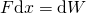,

essentially saying that “force times a small displacement equals the change in energy of some kind”. As I said, not very helpful for those calculus-averse.

Thus, here’s how I recommend approaching the topic: Imagine the rotor is moving/rotating at a constant speed. Then, compute the instantaneous mechanical power flowing into the moving object, and divide it by the velocity to obtain the force or torque.

Or, in equation form, divide both sides by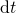, to get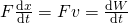,

saying that mechanical power equals the power coming from the change in energy.

As to how to exactly do this, there are two main approaches. I’ll mainly focus on reluctance networks here, but the methods apply just as well to actual field problems with B and H.

### Magnetic energy approach

The first approach is very straightforward, based on magnetic energy, the energy stored in the magnetic field. Let thr rotor move, and see how much the magnetic energy changes. Then, say all that energy goes to mechanical power and tadah – you have your force or torque.

Of course, the main assumption here is that the energy really goes to mechanical power. That means all the losses are ignored, and no power is being fed to the system. Typically, achieving this requires access to the model – the set of equations – itself.

For reluctance networks, using the energy approach requires that the fluxes stay constant everywhere. (Strictly speaking, this would often require changing the mmf sources, but in practice it matters not.)

With this assumption, the change in magnetic energy can be computed simply from the derivatives of the reluctances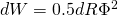.

Normally, this means derivating some airgap reluctances with respect to position, but that’s it. The rest of the problem can be ignored for now.

##### Physical interpretation

With purely physical quantities, the magnetic energy approach means that the flux density is kept constant. Simple as that.

Correspondingly, input currents may have to change to achieve this. However, since no voltage is induced anywhere, this does not lead to electric power flow – unlike the next approach.

### Magnetic coenergy approach

Another common approach is based on something called magnetic coenergy. While the energy approach maintained the fluxes (or B), the coenergy method keeps the mmfs (H) constant and lets fluxes (B) change. Otherwise, the approach is almost the same.

Not exactly, mind you. A changing flux means a voltage can be induced in any windings of the model. Since we also have currents, this means that power is flowing into the system, or out from it. Thus, we have to consider this term too, and not just the change of the energy stored in the field.

So, why use this more complex approach? With direct access to the model quantities and equations, the energy approach indeed rules. However, sometimes defining the “derivative of an airgap reluctance” can be a tad difficult. Especially if you’re dealing with a finite element model, for instance. In that case, you essentially have derivatives of a geometry that you gotta deal with. Coulomb did that, as far as I understand, but he’s got a huge name to live up to. Things are different for Smiths and Lehikoinens.

Point being, in some cases, the coenergy approach can be the only option. Simply do two simulations with the same currents but slightly different positions, and compute the differences in fluxes and energies. Not very pretty, but simple and practical.

### Power balance

Often, we’re not interested in instantaneous torque – only in average. In those cases, the power balance approach works nicely. Just calculate the average input power minus losses, and divide it by the speed.

An astute reader may now realize that this is a special case of the coenergy approach. Indeed, the average energy of the magnetic field is constant, leaving only the input/output terms.

The power balance approach works nicely with the simple airgap models. You only need to calculate the induced voltage, and multiply that with the currents the get the power. However, there are several options for how to exactly do this.

Note: here we assume that the airgap flux density is known. To achieve this, any of the already-described models can be used.

The straightworward way is to simply compute the flux linkage of the coils, and then take its derivative. (Thanks to our simple model, this can be done by an integration over the airgap surface.) This is the very definition of induced voltage, but can be tedious to achieve in practice if the airgap flux density has a complex waveform.

Things get considerably simpler if our currents are sinusoidal. In that case, only the first harmonic component of the flux density will generate a non-zero average power. In other words, if we have calculated the Fourier series presentation of the airgap flux density, we can drop all terms except for the one that has the correct number of poles and frequency.

##### Motion-induced EMF

There is also another approach. Indeed, we can assume that the airgap flux is stationary, while the stator is rotating around the rotor. Then, we can calculate the voltage induced to any individual conductor from the Lorentz term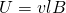or motional electromotive force.

However, be careful with the velocity. It refers to the relative velocity between the flux density wave, and the stator surface. Thus, for nonsinusoidal flux densities, the velocity can be – and usually is – different for different harmonics.

##### Mix-and-match

Finally, we don’t have to use individual conductors in the above approach. We can also compute the integral of the Lorentz-voltage and the equivalent surface current density. U times I equals P (or integral of E times J in this case, to be exact), after all.

Again here, we can use the full waveforms, or restrict our analysis to the fundamental harmonics. Or, we can use the full waveform of one of the quantities, and only the fundamental of the other. or vice versa.

No matter the combination, all approaches should yield the same result. Or, if they don’t, you know you’re making an error of some kind.

### Conclusion

Torque (and forces) can be reliably computed from the energy flow in your problem. This can be done

• Instantaneously, by directly derivating quantities in your model.
• By using small displacements and taking a numerical derivative.
• Looking at average values over one supply period.

Need help with electric motor design or design software? Let's get in touch - satisfaction guaranteed!Options for (Analytical) Torque Computation – Part 3